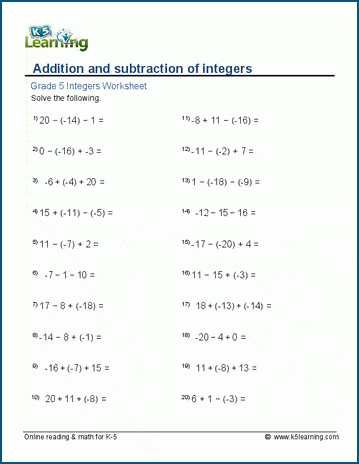# Integers Worksheets

## Integers & negative numbers

Our integers worksheets introduce concepts and operations related to negative numbers.

• Absolute values
• Comparing integers
• Integers and number lines
• Addition and subtraction of integers
• Multiplication and division of integers

• Addition & subtraction of integers
• Missing addend questions with integers
• Multiplication & division of integers
• Missing factor questions with integers

## Related topics

Order of OperationsSample Integers Worksheet

What is K5?

K5 Learning offers free worksheets, flashcards and inexpensive workbooks for kids in kindergarten to grade 5. Become a member to access additional content and skip ads.